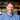# Simple Way To Swap Variables Using Javascript

May 24, 2019

I’ve been learning about some of the ways you can use destructuring to make my Javascript more declarative and communicate my intent more clearly.

One of the ways that I discovered was applying destructuring to swapping variables. This jumped out because last year I learned about bitwise operators and how to use them to swap variables without using temporary variables.

``````let swap = (i, j) => {
let temp = i
i = j
j = temp
}``````

We used this:1

``````var bitwiseSwap = (x, y) => {
if (x !== y) {
x = x ^ y
y = x ^ y
x = x ^ y
}
return { x, y }
}``````

``````var x = 10
var y = 20
console.log(bitwiseSwap(x, y)) // {x: 20, y: 10}``````

Destructuring offers an even simpler and more powerful approach to swapping variables and it too does not require temporary assignments.

# How To Use Destructuring To Swap Variables

To do the same thing with destructuring, it’s one line. No need for a swap function at all.

``````var x = 10
var y = 20
;[x, y] = [y, x]
console.log({ x, y }) // {x: 20, y: 10}``````

So what’s actually going on behind the scenes?

We’re declaring that we’d like to assign to x and y (the left hand side targets, `[x, y] =`) the values of the array with y in the 0th index position and x in the 1st index position (`[y, x]`) .

Javascript will execute the code and make the assignments.

# The Power Of Destructuring

Whereas bitwise operators are effective for integers, they will fall short with more complex data structures.

Imagine, instead of swapping 10 and 20, we wanted to swap an integer with an array (or two arrays, or arrays and objects, etc.) .

We would not be able to use our bitwise operators in this case. Here’s an example:

``````var x = 10
var z = [1, 2, 3]
console.log(bitwiseSwap(x, z)) // {x: 0, z: 10}``````

Using destructuring, however, things work much more smoothly.

``````var x = 10
var z = [1, 2, 3]
;[x, z] = [z, x]
console.log({ x, z }) // {x: [1, 2, 3], z: 10}``````

I love examples like this because they’re simple demonstrations of how Javascript is continuing to evolve and how there’s always new stuff to learn.

1 The function is the same in spirit, though it’s been modified slightly for clarity.Thanks for reading! My name's Stephen Weiss. I live in Chicago with my wife, Kate, and dog, Finn.